### Ball bouncing on a flexible...

This post discusses a solution for modeling a traveling load on Maplesim's Flexible Beam component and provides an example of a bouncing load.The idea for the above example came from an attempt to reproduce a model of a mass sliding on a beam from MapleSim's model gallery. However, reproducing it using contact components in combination with the Flexible Beam component turned out to be not straightforward, and this will be discussed in the following.

To simulate a traveling load on the Flexible Beam component, one could apply forces at discrete locations for a certain duration. However, the fidelity of this approach is limited by the number of discrete locations, which must be defined using the Flexible Beam Frame component, as well as the way in which the forces are activated.

One potential solution to address the issue of temporal activation of forces is to attach contact elements (such as Rectangle components) at distinct locations along the beam, which are defined by Flexible Beam Frame components, and make contact using a spherical or toroidal contact element. However, this approach also introduces two new problems:

• An additional bending moment is generated when the load is not applied at the center of the contact element's attachment point, the Flexible Beam Frame component. Depending on the length of the contact element, deformations caused by this moment can be greater than the deformation caused by the force itself when the force is applied at the ends of the contact element. Overall, this unwanted moment makes the simulation unrealistic and must be avoided.
• When the beam bends, a gap (see below) or an overlap is created between adjacent Rectangle components. If there is a gap, the object exerting a force on the beam can fall through it. Overlaps can create differences in dynamic behavior when the radius of curvature of the beam is on the opposite side of the point of contact.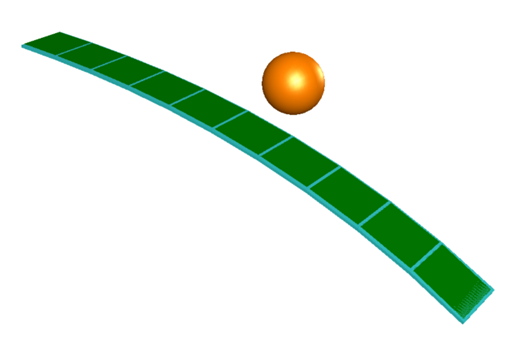To avoid these problems, the solution presented here uses an intermediate kinematic chain (encircled in yellow below) that redistributes the contact force on the Rectangle component on two support points (ports to attach Flexible Beam Frame components) in a linear fashion.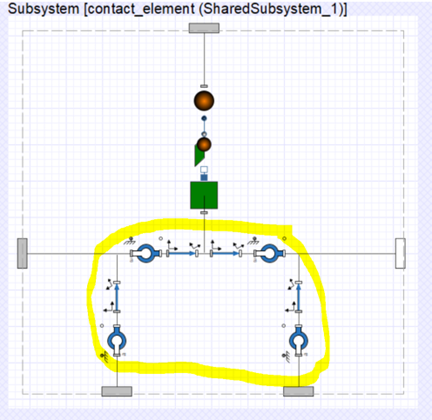To address gaps, the contact element (Rectangle) attached to the kinematic chain has the same width as the chain and connects to the adjacent contact elements via multibody frames. In the image below, 10 contact elements are laid on top of a single Flexible Beam component, like a belt made out of tiles. The belt has to be pinned to the flexible beam at one location (highlighted in yellow). The location of this fixed point determines how the flexible beam is loaded by tangential contact forces (friction forces) and should be selected carefully.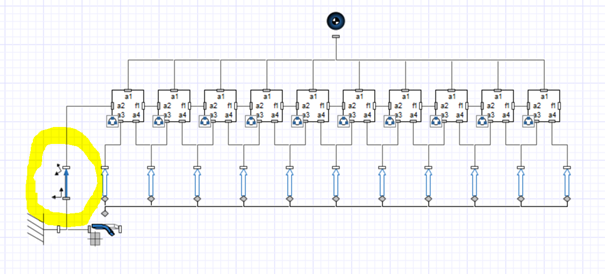Some observations on the attached model:

• Low damping and high initial potential energy of the ball can result in a failed simulation (due to constraint projection failure). Increasing the number of elastic coordinates has a similar effect. Constraint projection can be turned off in the simulation settings to continue simulation.
• The bouncing ball excites several eigenmodes at once, causing the beam to wiggle chaotically in combination with the varying bouncing frequency of the ball. A similar looking effect can also be achieved with special initial conditions, as demonstrated with Maple in this excellent post on Euler beams and partial differential equations.
• Repeated simulations with low damping lead to different results (an indication of chaotic behavior; see three successive simulations below (gold) compared to a saved solution(red)). The moment in the animation when the ball travels backward represents a metastable equilibrium point of the simulation. This makes predictions beyond this point difficult, as the behavior of the system is highly dependent on the model parameters. Whether the reversal is a simulation artifact or can happen in reality remains to be seen. Overall, this example could evolve into a nice experimental fun project for students.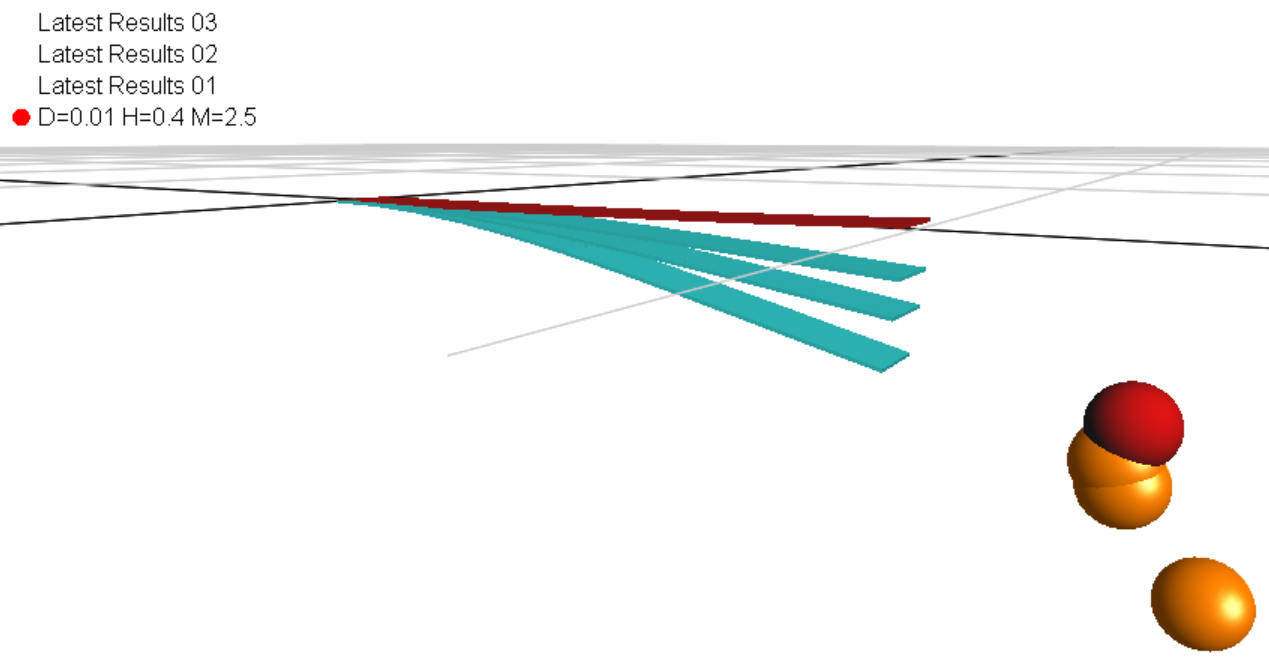• Setting the gravitational constant for Mars, everything is different. I could not reproduce the fun factor on Earth. A reason more to stay ,-)

Ball_bouncing_on_a_flexible_beam.msim

### New Proof of The Pythagorean...

In March of 2023, two high school students, Calcea Johnson, and Ne’Kiya Jackson, presented a new proof of the Pythagorean Theorem at the American Mathematical Society’s Annual Spring Southeastern Sectional Meeting. These two young women are challenging the conventions of math as we know it.
The Pythagorean Theorem states that in a right angle triangle, the sum of the squares of the legs is equal to the square of the hypotenuse: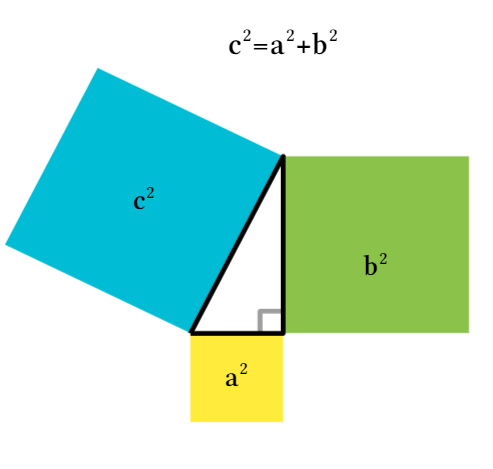The theorem has been around for over two thousand years and has been proven hundreds of times with many different methods. So what makes the Johnson-Jackson proof special? The proof is one of the first to use trigonometry.
For years, mathematicians have been convinced that a trigonometric proof of the Pythagorean Theorem is impossible because much of trigonometry is based upon the Pythagorean Theorem itself (an example of circular reasoning).
That said, some results in trigonometry are independent of the Pythagorean Theorem, namely the law of sines, and the sine and cosine ratios; the latter is a result that 12-year-old Einstein used in his trigonometric proof of the theorem.
Though all the details of the Johnson-Jackson proof have not been made public, there was enough information for me to recreate the proof in Maple Learn. The idea of the proof is to construct a right angle triangle with an infinite series of congruent right angle triangles (the first of which has side lengths a, b, and c). Then, using the sine ratio, solve for the hypotenuse lengths of each small congruent triangle. To explore this construction see Johnson and Jackson’s Triangle Construction on Maple Learn.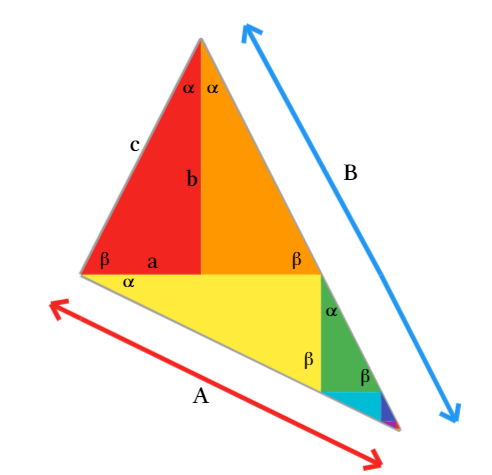Next, find the side lengths of the large triangle (A and B) by evaluating an infinite sum (composed of the hypotenuse lengths of the small congruent triangles). Finally, apply the law of sines to the isosceles triangle made from the first 2 congruent triangles. After simplifying this expression, the Pythagorean relationship (c2 = a2 + b2) emerges.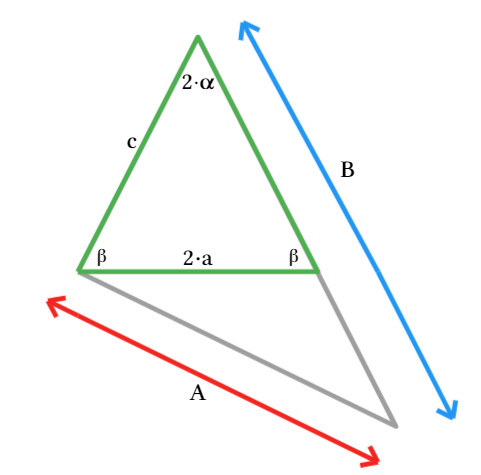To see more details of the proof, check out Johnson and Jackson’s Proof of Pythagorean Theorem on Maple Learn.
This new proof of the Pythagorean Theorem shows that discoveries in math are still happening and that young people can play a big role in these discoveries!

## invalid input: simplify/Jacobi/JacobiDN expects...

Maple 2023 asked by May 25

## Why do I get this sequence returned from alias...

Maple 2023 asked by May 25